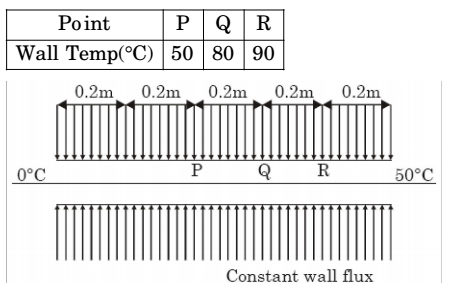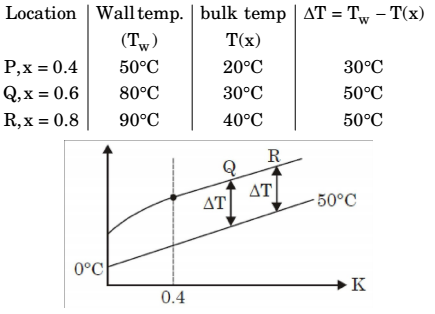## Heat Transfer Miscellaneous

#### Heat-Transfer

1. The Blausius equation related to boundary layer theory is a

1. Blausius equation:

 2d3f + fd²f = 0 dη3 dη²

This order non-linear D.E.

##### Correct Option: D

Blausius equation:

 2d3f + fd²f = 0 dη3 dη²

This order non-linear D.E.

1. For flow of viscous fluid over a flat plate, if the fluid temperature is the same as the plate temperature, the thermal boundary layer is

1. NA

##### Correct Option: D

NA

1. Water flows through a tube of diameter 25 mm at an average velocity of 1.0 m/s. The properties of water are ρ = 1000 kg/m3, μ= 7.25 × 104 Ns/m², k = 0.625 W/mK, Pr = 4.85. Using Nu = 0.023 Re0.8 Pr0.4, the convective heat transfer coefficient (inW/m²K) is _______

1.  Re = ρvd μ

 = 1000 × 1 × 25 × 103 7.25 × 10-4

= 34482.75
Pr = 4.85
Nu = 0.023 Re0.8 Pr0.4.
Nu = .0.23 × (34482.758)0.8 (4.85)0.4.
= 184.546
 &Nu = hd k

 h = Nu.k d

 = 184.546 × 0.625 25 × 10-3

 = 4613.66 W m²K

##### Correct Option: A

 Re = ρvd μ

 = 1000 × 1 × 25 × 103 7.25 × 10-4

= 34482.75
Pr = 4.85
Nu = 0.023 Re0.8 Pr0.4.
Nu = .0.23 × (34482.758)0.8 (4.85)0.4.
= 184.546
 &Nu = hd k

 h = Nu.k d

 = 184.546 × 0.625 25 × 10-3

 = 4613.66 W m²K

1. The wall of a constant diameter pipe of length 1 m is heated uniformly with flux q” by wrapping a heater coil around it. The flow at the inlet to the pipe is hydrodynamically fully developed. The fluid is incompressible and the flow is assumed to be laminar and steady all through the pipe. The bulk temperature of the fluid is equal to 0°C at the i nlet and 50°C at the exi t. The wall temperatures are measured at three locations, P, Q and R as shown in the figure. The flow thermally develops after some distance from the inlet. The following measurements are made:Among the locations P, Q and R, the flow is thermally at:

1. I n case of uni for m heat flux, bulk mean t emperat ur e var ies linearly.
The difference bet ween bulk mean temperature and wall temperature is constant in thermally developed region so bulk tempeature,
T(x) = A + Bx
At x = 0, T = 0°C
⇒ A = 0°C
At x = 1, T = 50°C
⇒ B = 50°C
∴ T(x) = 50x
Here,So, Q and R in thermally developed region.

##### Correct Option: D

I n case of uni for m heat flux, bulk mean t emperat ur e var ies linearly.
The difference bet ween bulk mean temperature and wall temperature is constant in thermally developed region so bulk tempeature,
T(x) = A + Bx
At x = 0, T = 0°C
⇒ A = 0°C
At x = 1, T = 50°C
⇒ B = 50°C
∴ T(x) = 50x
Here,So, Q and R in thermally developed region.

1. For a hydrodynamically and ther mally fully developed laminar flow through a circular pipe of constant cross-section. The Nusselt number at constant wall heat flux (Nuq) and that at constant wall temperature (NuT) are related as

1. (Nu)q for constant wall heat flux and (Nu)T at constant wall temperature for a hydrodynamically and t hermally fully developed laminar flow through a circular pipe of constant cross-section is 4.36 and 3.66 respectively. Hence, (Nu)q > (Nu)T

##### Correct Option: D

(Nu)q for constant wall heat flux and (Nu)T at constant wall temperature for a hydrodynamically and t hermally fully developed laminar flow through a circular pipe of constant cross-section is 4.36 and 3.66 respectively. Hence, (Nu)q > (Nu)T